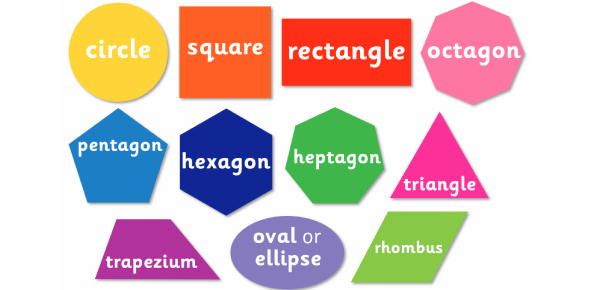# 4th Grade Math Questions On 2D Shapes! Trivia Quiz

16 Questions | Attempts: 9825SettingsThis trivia quiz is made up of 4th grade math questions on 2d shapes! There are a lot of people who get confused when it comes to solving math problems which involve different shapes and sizes and if you are among them, worry not as the quiz below will help refresh your memory on the basics. How about you give it a try and see how well you will do. Good luck!

• 1.
Which of the following is a seven-sided polygon?
• A.

Triangle

• B.

Heptagon

• C.

Hexagon

• D.

Octagon

• E.

Acute Triangle

• 2.
What is the measurement of a right angle?
• A.

30 degrees

• B.

45 degrees

• C.

90 degrees

• D.

180 degrees

• E.

360 degrees

• 3.
Which of the following angle measurements is NOT an obtuse angle?
• A.

100 degrees

• B.

180 degrees

• C.

45 degrees

• D.

95 degrees

• E.

360 degrees

• 4.
Which of the following is a polygon whose sides are all the same length, and whose angles are all the same?
• A.

Triangle

• B.

Heptagon

• C.

Acute Triangle

• D.

Regular Polygon

• E.

Octagon

• 5.
A shape that contains only one pair of pararellel sides.
• A.

Rhombus

• B.

Trapezoid

• C.

Square

• D.

Triangle

• 6.
A shape that has four equal sides and opposite angles equal.
• A.

Square

• B.

Rhombus

• C.

Both a and b

• D.

Neither a or b

• 7.
A shape that has four congruent sides and four right angles.
• A.

Square

• B.

Rectangle

• C.

Rhombus

• D.

Trapezoid

• 8.
A shape that has one right angle.
• A.

Acute triangle

• B.

Obtuse triangle

• C.

Equilateral triangle

• D.

Right triangle

• 9.
All squares are rectangles.
• A.

True

• B.

False

• 10.
All quadrilaterals have one pair of parallel sides.
• A.

True

• B.

False

• 11.
All rectangles are squares.
• A.

True

• B.

False

• 12.
A triangles with all three sides equal is called _____________.
• 13.
A triangle with only two sides equal is called an ________________.
• 14.
The sum of the angles in a triangle is ___________.
• 15.
The sum of the four angles in a quadrilateral is ______________.
• 16.
Each angle in an equilateral triangles measures _____________.

## Related TopicsBack to top
×

Wait!
Here's an interesting quiz for you.